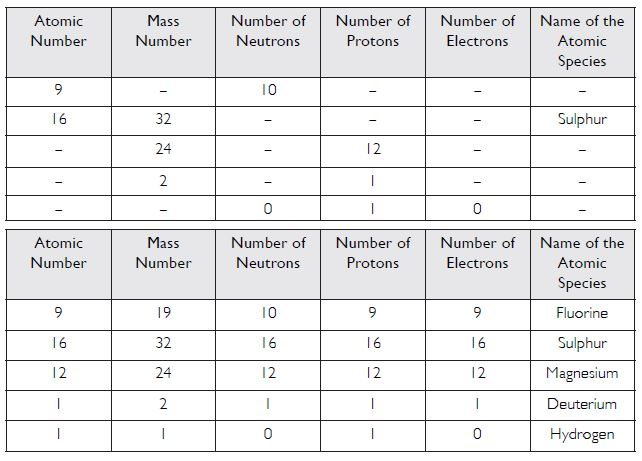# NCERT Solutions for Class 9 Science Chapter 4 - Structure of Atom

##### Question 1:

What are canal rays?

Rays or streams of positive particles, which travel in a direction from the anode to the cathode, when a gas taken in a discharge tube is subjected to the action of high voltage under low pressure is called canal rays.

##### Question 2:

If an atom contains one electron and one proton, will it carry any charge or not?

No, it will not carry any charge.

##### Question 3:

On the basis of Thomson’s model of an atom, explain how the atom is neutral as a whole.

(1) According to Thomson, an atom may be regarded as a uniform sphere of positive electricity (protons) in which negatively charged electrons are embedded like the seeds in a watermelon.

##### Question 4:

On the basis of Rutherford’s model of an atom which sub-atomic particle is present in the nucleus of an atom?

Proton

##### Question 5:

Draw a sketch of Bohr’s model of an atom with three shells.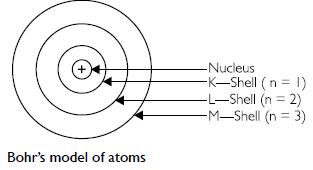##### Question 6:

What do you think would be the observation if the alpha-particle scattering experiment is carried out using a foil of metal other than gold?

In such a case, almost all the alpha-particles would pass without hitting the atoms and hardly any alpha particle would be deflected.

##### Question 7:

Name the three sub-atomic particles of an atom.

(a) Electron (b) Proton (c) Neutron

##### Question 8:

Helium atom has an atomic mass of 4 u and two protons in its nucleus. How many neutrons does it have?

Atomic mass of Helium = 4u
No. of protons = 2
$\therefore$ No. of neutrons = 4 - 2 = 2

##### Question 9:

Write the distribution of electrons in carbon and sodium atoms.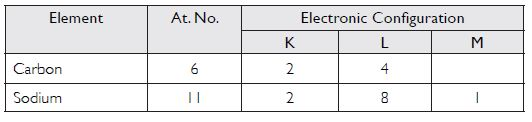##### Question 10:

If K and L shells of an atom are full, then what would be the total number of electrons in the atom?

No. of electrons in K-shell = 2
No. of electrons in L-shell = 8
Total no. of electrons = 2 + 8 = 10

##### Question 11:

How will you find the valency of chlorine, sulphur and magnesium?

Chlorine has the electronic configuration = 2, 8, 7
$\therefore$ Valency of chlorine = 8 – 7 = 1
Sulphur has the electronic configuration = 2, 8, 6
$\therefore$ Valency of sulphur = 8 – 6 = 2

##### Question 12:

If the number of electrons in an atom is 8 and number of protons is also 8, then
(i) What is the atomic number of the atom? and
(ii) What is the charge on the atom?

(i) No. of protons = 8
$\therefore$ Atomic no. of the element = 8
(ii) Zero

##### Question 13:

With the help of the table given below, find out the mass number of oxygen and sulphur atom.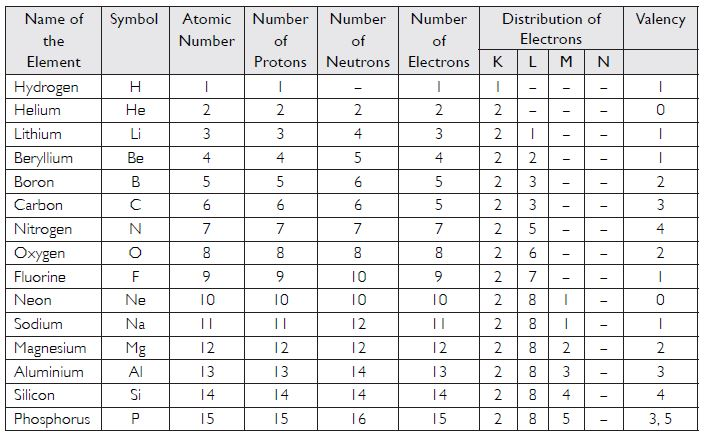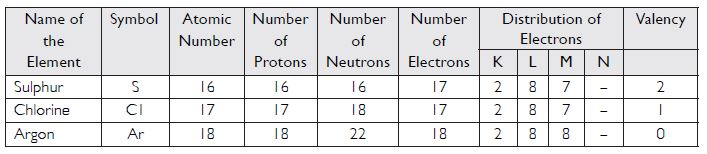Mass number of oxygen = 8 + 8 = 16
Mass number of sulphur = 16 + 16 = 32

##### Question 14:

For the symbol H, D and T, tabulate three sub-atomic particles found in each of them.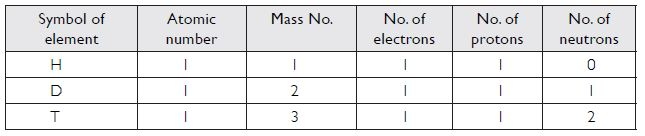##### Question 15:

Write the electronic configuration of any one pair of isotopes and isobars.

Pair of isotopes :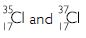Atomic number of Cl = 17

##### Question 16:

Compare the properties of electrons, protons and neutrons.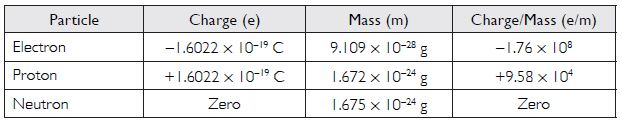##### Question 17:

What are the limitations of J. J. Thomson’s model of the atom?

1. It could not explain the observations of Rutherford’s alpha-particle scattering experiment.
2. It could not explain the hydrogen spectrum.

##### Question 18:

What are the limitations of Rutherford’s model of the atom?

1. It can’t explain the stability of the atom.
2. It can’t explain the spectrum of hydrogen.

##### Question 19:

Describe Bohr model of the atom.

##### Question 20:

Compare all the proposed models of an atom given in this chapter text.

The proposed models of atoms discussed in this chapter text are Thomson’s model, Rutherford’s model and Bohr’s model of atom.
A. Thomson’s model of an atom
1. According to Thomson, an atom may be regarded as a uniform sphere of positive electricity (protons) in which negatively charged electrons are embedded like the seeds in a watermelon.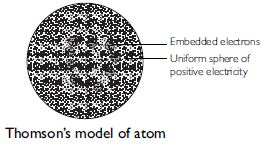2. The total positive charge is equal to the total negative charge of all the electrons so that the atom on the whole is electrically neutral.
B. Rutherford’s nuclear model of an atom
1. Atom has a dense, heavy, positively charged central part called nucleus.
2. The electrons are present at very large distances from the nucleus.
3. The total positive charge on the nucleus is equal to the total negative charge of all the electrons such that the atom on the whole is electrically neutral.
4. The electrons are revolving around the nucleus and the attractive force of the nucleus is balanced by the centrifugal force (just like the planets revolving around the sun).
5. It is different from Thomson’s model, because in Thomson’s model, the total mass, positive charge and electrons are uniformly distributed in an atom.
C. Bohr’s model of an atom
1. An atom has three types of particles called fundamental particles. These are electrons, protons and neutrons. The protons and neutrons are present in the nucleus, which is present at the centre of the atom. The electrons are present around the nucleus and are at a very large distance from it. There is a large vacant space between the nucleus and the electrons.
2. An atom is electrically neutral as in an atom, the number of protons; each with one positive charge is equal to the number of electrons; each with one negative charge.
3. The electrons are revolving around the nucleus in fixed circular paths which are called energy levels or energy shells or orbits. The energy levels or energy shells can be counted in two ways 1, 2, 3, 4, 5, 6 or as K, L, M, N, O, P. The counting starts outwards from the centre.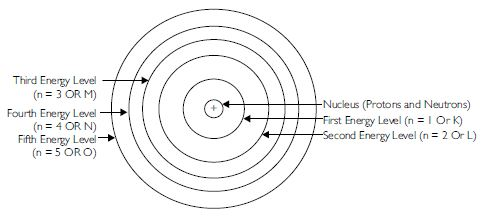4. The various energy levels are arranged in order of increasing energy. The order of energy is
1 < 2 < 3 < 4 < 5...........or K < L < M < N < O.............
5. The energy of an electron in an atom is quantised.
6. There is no change in the energy of the electrons as long as they keep on revolving in the same energy level.
7. The change in energy takes place only when the electron jumps from one energy level to the other. It gains energy when it jumps from a lower energy level to a higher energy orbital. On the other hand, it loses energy when it jumps from a higher energy level to a lower energy level.
8. The angular momentum of an electron in an atom is also quantised.

##### Question 21:

Summarise the rules for writing distribution of electrons in various shells for the first eighteen elements.

(i) The maximum number of electrons present in a shell is given as 2n2, where ‘n’ is the orbit number. For example:
For K shell, n = 1
Maximum number of electrons = 2n2 = 2 x (1)2 = 2
For L shell, n = 2,
Maximum number of electrons = 2n2 = 2 x (2)2 = 8
(ii) Electrons cannot be accommodated in a shell, unless inner shells are completely filled.
(iii) The maximum number of electrons that can be accommodated in the outermost shell is 8.

##### Question 22:

Define valency by taking examples of silicon and oxygen.

Valency is the combining capacity of an atom of an element. It is numerically equal to the number of valence electrons, in case the number of valence electrons is 1 to 4. On the other hand, it is equal to eight minus the number of valence electrons, in case the number of valence electrons is more than 4.

Silicon (14Si) has electronic configuration =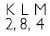$\therefore$ Valency = No. of valence electrons = 4
Oxygen (8O) has electronic configuration = 2, 6
$\therefore$ Valency = 8 – number of valence electrons = 8 – 6 = 2

##### Question 23:

Explain with examples (i) Atomic number (ii) Mass number (iii) Isotopes (iv) Isobars. Give any two uses of Isotopes.

(i) Atomic number: It is the number of protons present in the nucleus of an atom of an element.
(ii) Mass number: It is the sum of total number of protons and neutrons present in the nucleus of an atom of an element.
(iii) Isotopes: These are the atoms of the same element having same atomic number but different mass numbers.
(iv) Isobars: These are the atoms of different elements having the same mass number but different atomic numbers.
Uses of isotopes

1.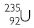isotope of uranium is used as a fuel in nuclear reaction.

2.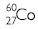isotope of cobalt is used in the treatment of cancer.

##### Question 24:

Na+ has completely filled K and L shells. Explain.

Atomic number of Na = 11
$\therefore$ No. of electrons in Na = 11
No. of electrons in Na+ = 11 – 1 = 10
The electronic configuration of Na+ = 2, 8 K L
$\therefore$ In Na+, both K and L shells are completely filled.

##### Question 25:

If bromine atom is available in the form of say two isotopes Br (49.7%) and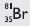.(50.3%), calculate the average atomic mass of bromine atom.

1. The two isotopes of bromine are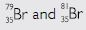(49.7%) (50.3%)
Average atomic mass of bromine atom =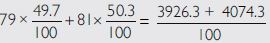=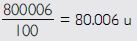##### Question 26:

The average atomic mass of a sample of an element X is 16.2 u, what are the percentages of isotopes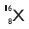and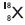Let % age of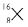.= p

% age of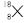.= 100 – p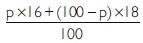. = 16.2

16 p – 18 p + 1800 = 1620
– 2p = – 180
p = 90

\ % age of. = 90 %

\ % age of. = 100 – 9010 = 10 %

##### Question 27:

If Z = 3, what would be the valency of the element? Also, name the element.

Z = 3
\ Atomic no. of element = 3

Its electronic configuration =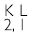Its valency = 1 (No. of electrons in the valence shell.)
Name of the element with atomic number 3 and valency 1 is lithium.

##### Question 28:

Composition of the nuclei of two atomic species X and Y are given as under:
X Y
Protons 6 6
Neutrons 8 8
Give the mass numbers of X and Y. What is the relation between the two species?

Mass no. of X = 6 + 6 = 12
Mass no. of Y = 6 + 8 = 14
The two species are isotopes because they have the same atomic number but different mass numbers.

##### Question 29:

For the following statements write T for True and F for False.
(a) J. J. Thomson proposed that the nucleus of an atom contains only nucleons.
(b) A neutron is formed by an electron and a proton combining together. Therefore, it is neutral.

(c) The mass of an electron is about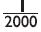times that of a proton.

(d) An isotope of iodine is used for making tincture iodine, which is used as a medicine.

(a) F (b) F (c) T (d) F

##### Question 30:

Rutherford’s alpha-particle scattering experiment was responsible for the discovery of:
(a) atomic nucleus (b) electron
(c) proton (d) neutron

(a) Atomic nucleus (✓)
(b) Electron (x)
(c) Proton (x)
(d) Neutron (x)

##### Question 31:

Isotopes of an element have:
(a) the same physical properties.
(b) different chemical properties.
(c) different number of neutrons.
(d) different atomic numbers.

(a) the same physical properties. (✓)
(b) different chemical properties (x)
(c) different number of neutrons. (✓)
(d) different atomic numbers. (x)

##### Question 32:

Number of valence electrons in Cl ion are (a) 16 (b) 8 (c) 17 (d) 18

(a) 16 (x) (b) 8 (✓) (c) 17 (x) (d) 18 (x)

##### Question 33:

Which one of the following is a correct electronic configuration of sodium?
(a) 2, 8 (b) 8, 2, 1 (c) 2, 1, 8 (d) 2, 8, 1